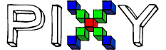# Block Signature question using pixy.ccc.numBlocks

Hello, I am a student that is relatively new to coding with an Arduino combined with the pixy camera, so I’m not sure if this question has been answered already or not. I currently have a code similar to the one below (I am not able to share my exact code, so this was borrowed from another forum post that was linked at the bottom). I have tried to implement if statements nested within the for loop so that my robot will do a specific task based on the block that the pixy recognizes. However, when I try to use the pixy.ccc.blocks[i].m_signature command and print the signatures, the first block in the frame (regardless of color) outputs as a signature zero. When there are 2 blocks in the frame, it outputs a zero and a one, and so on. I want to be able to have each block associated with a specific number so I can use an if statement similar to…

“if (pixy.ccc.blocks[i].m_signature == 1)” and “if (pixy.ccc.blocks[i].m_signature == 2)” , etc.

I would appreciate any help, and if this is confusing, I can try to explain using my whole code once I figure out how to upload it on the forums correctly.

Thank you,

Similar Code:

``````void loop()
``````

{
int i;
// grab blocks!
pixy.ccc.getBlocks();

// If there are detect blocks, print them!
if (pixy.ccc.numBlocks)
{
Serial.print("Detected “);
Serial.println(pixy.ccc.numBlocks);
for (i=0; i<pixy.ccc.numBlocks; i++)
{
Serial.print(” block “);
Serial.print(i);
Serial.print(”: ");
pixy.ccc.blocks[i].print();

``````}
``````

}
}

Credit: Edge (Edward)

Hi Keagan,

that’s odd — the m_signature variable should range from 1 to 7, which represent Pixy’s 7 different color signatures you can teach and track.

Quick info on the Arduino API here: https://docs.pixycam.com/wiki/doku.php?id=wiki:v2:hooking_up_pixy_to_a_microcontroller_-28like_an_arduino-29#arduino-api

Hope this helps!

Cheers,
Jesse